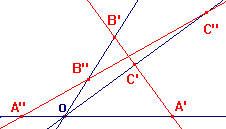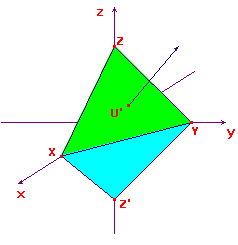betweenness and other matters

 In euclidean, similarity and affine geometry, we have the concept of betweenness. Given three collinear points, one lies between the other two. This is preserved by the appropriate transformations. The concept of line segment is based on betweenness. In projective geometry, there is no corresponding concept. Suppose that A,B,C are collinear p-points. They are represented by three coplanar lines through O. Imagine that they lie in the plane of the screen. In a general embedding, the representatives of A,B,C are collinear points. In the sketch, these are the red lines A'B'C' and A"B"C". Observe that C' lies between A' and B', while B" lies between A" and C". Thus, there is no sensible way to say that one of A,B,C lies between the other two.It follows that we cannot talk of the segment of a p-line defined by two p-points on the p-line. As a consequence, the definition of a triangle in projective geometry is rather different from that in the other geometries. If A,B,C are non-collinear p-points, then the sides of ΔABC are the (complete) p-lines AB, BC and CA. Given three distinct p-lines, L,M,N, these define a triangle with vertices the intersections of the p-lines in pairs. Another concept which can be defined in terms of betweenness is that of the interior of a triangle. The sides of a projective triangle are represented by three planes through O. These divide space into eight regions. There is no consistent way to choose any one of these as the interior. example Consider the projective triangle Δ with vertices [1,0,0], [0,1,0] and [0,0,1]. These are representd by the coordinate axes. The sides are represented by the coordinate planes. The point P[1,1,1] does not lie on any side of the triangle. We shall look at the embedding in two different planes. Let Π be the plane x+y+z = 1, and Φ the plane x+y-z = 1. In Π, the vertices embed as X(1,0,0), Y(0,1,0), Z(0,0,1). A simple calculation shows that P embeds as U'(1/3,1/3,1/3). This lies in the triangle XYZ shown in green in the sketch. In Φ, the vertices embed as X, Y and Z'(0,0,-1). By direct calculation, P embeds as U(1,1,1). The triangle XYZ' on Φ (shown in cyan) does not contain U. On the other hand, we can define the interior of a projective conic in a way consistent with the projective group.